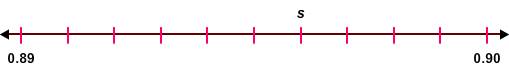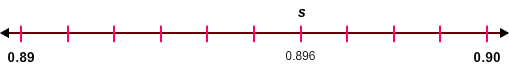# Decimal Number Lines Part 2

Rate 0 stars Common Core
Quiz size:
Message preview:
Someone you know has shared Decimal Number Lines Part 2 quiz with you:

To play this quiz, click on the link below:

https://www.turtlediary.com/quiz/decimals-on-number-lines.html

Hope you have a good experience with this site and recommend to your friends too.

Login to rate activities and track progress.
Login to rate activities and track progress.

Consider the number line given below.Let's find the decimal represented by the point s.

Observe that:

The distance between 0.89 and 0.90 is divided into 10 equal parts.

So, each part represents a length of one-thousandth or 0.001.

Now, count the number of parts from 0.89 to s.

There are 6 parts.

This means that the point s is 6 parts to the right of 0.89 or 6 thousandths after 0.89.

Now, 0.89 and 6 thousandths equals 0.896.

So, the decimal represented by the point s is 0.896.ds

A B C D E F G H I J K L M N O P Q R S T U V W X Y Z